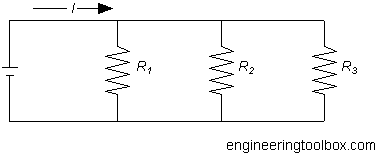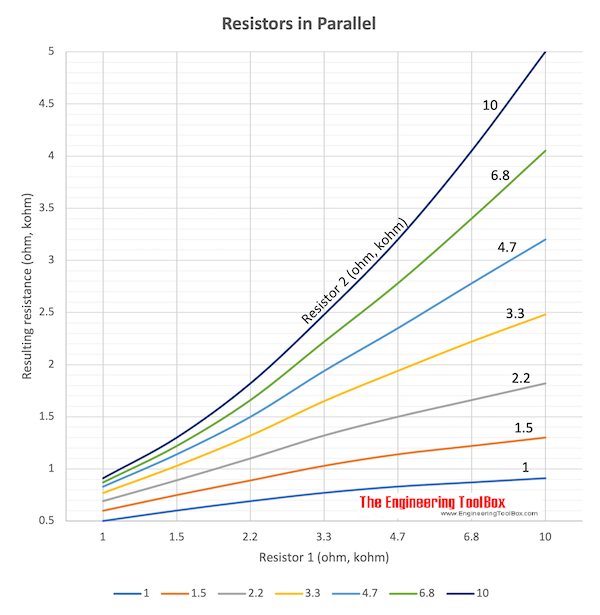Engineering ToolBox - Resources, Tools and Basic Information for Engineering and Design of Technical Applications!

# Electrical Parallel Circuits

## Resistance, voltage and current in electrical parallel circuits.

In a parallel circuit there is more than one path the electrons can flow.

The Parallel Circuit Rules:

1. The total current in parallel circuit is equal to the sum of the currents in all the branches of the circuit.
2. The total voltage across a parallel circuit is equal to the voltage across any of the branches in the circuit.
3. The total resistance of a parallel circuit is calculated by using Ohm's law on the voltage and current of the whole circuit.The sum of currents in a parallel network can be calculated as

I = I1 + I2 + ... + In             (1)

where

In = parallel current (amps)

I = sum of currents (amps)

The total resistance in a parallel circuit can be calculated as

1 / R = 1 / R1 + 1 / R2 + ... + 1 / Rn             (2)

where

Rn = parallel resistance (ohms, Ω)

R = total resistance in the parallel network (ohms, Ω)

### Example - Parallel Network

The total resistance in a network with three resistors R1 = 10 Ω, R2 = 20 Ω  and R3 = 30 Ω can be calculated as

1 / R = 1 / (10 Ω) + 1 / (20 Ω) + 1 / (30 Ω)

= 0.183 (1/Ω)

R = 1 / 0.183

= 5.46 (Ω)

Connected to a 12 V battery the sum of currents can be calculated

I = U / R

=  (12 V) / (5.46 Ω)

= 2.2 Amps)

The current through each resistor can be calculated as

I1= U / R1

=  (12 V) / (10 Ω)

= 1.2 (Amps)

I2= U / R2

=  (12 V) / (20 Ω)

= 0.6 (Amps)

I3= U / R3

=  (12 V) / (30 Ω)

= 0.4 (Amps)

### Resistors in Parallel and resulting Resistance

Two resistors in parallel - resistance ranging 1 - 100 ohm

 Resulting Resistance (Ω) R2(Ω) R1 (Ω) 1 1.5 2.2 3.3 4.7 6.8 10 15 22 33 47 68 1 0.5 0.6 0.69 0.77 0.83 0.87 0.91 0.93 0.95 0.97 0.98 0.99 1.5 0.6 0.75 0.89 1.03 1.14 1.22 1.30 1.36 1.40 1.43 1.45 1.46 2.2 0.69 0.89 1.1 1.32 1.50 1.66 1.82 1.92 2.0 2.06 2.10 2.13 3.3 0.77 1.03 1.32 1.65 1.94 2.22 2.48 2.70 2.87 3.00 3.08 3.14 4.7 0.83 1.14 1.50 1.94 2.35 2.78 3.20 3.58 3.87 4.12 4.27 4.39 6.8 0.87 1.22 1.66 2.22 2.78 3.40 4.05 4.68 5.19 5.64 5.94 6.18 10 0.91 1.30 1.82 2.48 3.20 4.05 5.0 6.0 6.9 7.7 8.3 8.7 15 0.93 1.36 1.92 2.70 3.58 4.68 6.0 7.50 8.9 10.3 11.4 12.2 22 0.95 1.40 2.00 2.87 3.87 5.19 6.9 8.9 11.0 13.2 15.0 16.6 33 0.97 1.43 2.06 3.0 4.12 5.64 7.7 10.3 13.2 16.5 19.4 22.2 47 0.98 1.45 2.1 3.08 4.27 5.94 8.3 11.4 15.0 19.4 23.5 27.8 68 0.99 1.46 2.13 3.14 4.39 6.18 8.7 12.2 16.6 22.2 27.8 34.0## Related Topics

• Electrical - Electrical units, amps and electrical wiring, wire gauge and AWG, electrical formulas and motors.

## Engineering ToolBox - SketchUp Extension - Online 3D modeling!

Add standard and customized parametric components - like flange beams, lumbers, piping, stairs and more - to your Sketchup model with the Engineering ToolBox - SketchUp Extension - enabled for use with the amazing, fun and free SketchUp Make and SketchUp Pro .Add the Engineering ToolBox extension to your SketchUp from the SketchUp Pro Sketchup Extension Warehouse!

Translate

## Privacy

We don't collect information from our users. Only emails and answers are saved in our archive. Cookies are only used in the browser to improve user experience.

Some of our calculators and applications let you save application data to your local computer. These applications will - due to browser restrictions - send data between your browser and our server. We don't save this data.

## Citation

• Engineering ToolBox, (2009). Electrical Parallel Circuits. [online] Available at: https://www.engineeringtoolbox.com/parallel-networks-d_1385.html [Accessed Day Mo. Year].

Modify access date.

. .

#### Scientific Online Calculator3 30